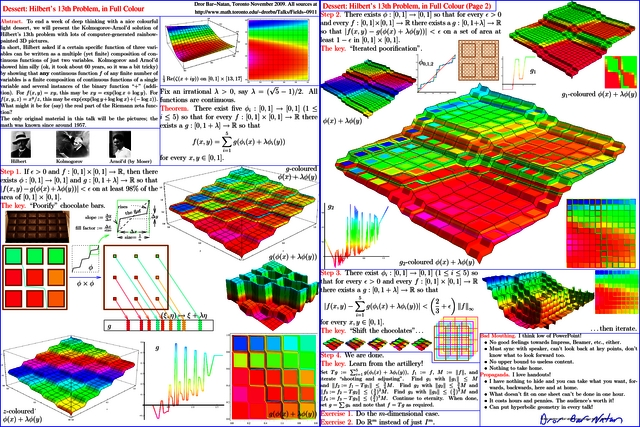© | << < ? > >> | Dror Bar-Natan: Talks:

# Dessert: Hilbert's 13th Problem, in Full Colour

### Workshop on Computational Differential Geometry, Topology, and DynamicsThe Fields InstituteSaturday November 29, 2009, 11AM

Abstract. To end a week of deep thinking with a nice colourful light dessert, we will present the Kolmogorov-Arnol'd solution of Hilbert's 13th problem with lots of computer-generated rainbow-painted 3D pictures.

In short, Hilbert asked if a certain specific function of three variables can be written as a multiple (yet finite) composition of continuous functions of just two variables. Kolmogorov and Arnol'd showed him silly (ok, it took about 60 years, so it was a bit tricky) by showing that ANY continuous function f of any finite number of variables is a finite composition of continuous functions of a single variable and several instances of the binary function "+" (addition). For f(x,y)=xy, this may be xy=exp(log x + log y). For f(x,y,z)=x^y/z, this may be exp(exp(log y + log log x) + (-log z)). What might it be for (say) the real part of the Riemann zeta function?

The only original material in this talk will be the pictures; the math was known since around 1957.Sources at Hilbert13th.zip and at Academic Pensieve: 2009-11.